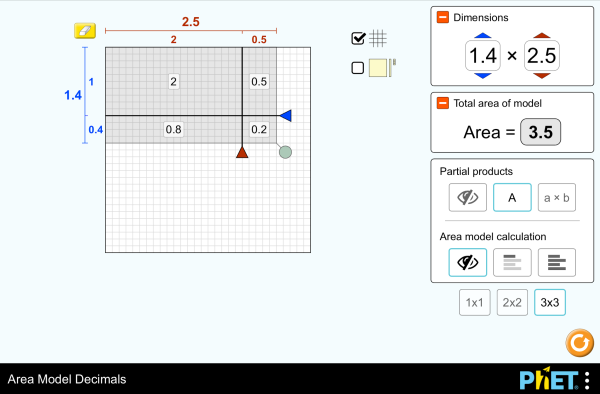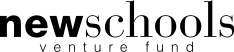# Area Model Decimals

Aceste simulări nu au fost încă traduse în limba română . Puteți accesa simularea, din meniul de mai jos, în limba engleză.DescarcăInclude Închide Include o copie functională a simulării Folosiţi acest cod sursă pentru a include o simulare. Puteţi mofifica dimensiunile prin modificarea parametrilor "width" şi "height". Include o imagine care lansează simularea
Rulează
Folosiţi acest cod sursă pentru afişarea "Rulează" Multiplicare Decimals Area Model PhET is supported byand educators like you.

### Subiecte

• Multiplicare
• Decimals
• Area Model
• Factors
• Partial Products

### Descriere

Build rectangles of various sizes and relate multiplication to area. Partition a rectangle into smaller areas and discover new strategies for multiplying decimals!

### Studii de caz

• Recognize that area represents the product of two numbers and is additive.
• Develop and justify a strategy that uses the area model to simplify a decimal multiplication problem.
• Devise a strategy to determine the product of two rational numbers (or an integer and a rational number) by representing the product as an area or the sum of areas.
• Use an area model to understand why multiplying a given number by a decimal less than 1 results in a product smaller than the given number.
• Interpret the product of two rational numbers using base-ten tiles

### Standards Alignment

#### Common Core - Math

5.NF.B.4
Apply and extend previous understandings of multiplication to multiply a fraction or whole number by a fraction.
5.NF.B.4a
Interpret the product (a/b) × q as a parts of a partition of q into b equal parts; equivalently, as the result of a sequence of operations a × q ÷ b. For example, use a visual fraction model to show (2/3) × 4 = 8/3, and create a story context for this equation. Do the same with (2/3) × (4/5) = 8/15. (In general, (a/b) × (c/d) = ac/bd.)
5.NF.B.4b
Find the area of a rectangle with fractional side lengths by tiling it with unit squares of the appropriate unit fraction side lengths, and show that the area is the same as would be found by multiplying the side lengths. Multiply fractional side lengths to find areas of rectangles, and represent fraction products as rectangular areas.
5.NF.B.5
Interpret multiplication as scaling (resizing), by:
5.NF.B.5a
Comparing the size of a product to the size of one factor on the basis of the size of the other factor, without performing the indicated multiplication.
5.NF.B.5b
Explaining why multiplying a given number by a fraction greater than 1 results in a product greater than the given number (recognizing multiplication by whole numbers greater than 1 as a familiar case); explaining why multiplying a given number by a fraction less than 1 results in a product smaller than the given number; and relating the principle of fraction equivalence a/b = (n × a)/(n × b) to the effect of multiplying a/b by 1.
Versiune 1.2.1

### Activități ale profesorilor

Games Remote Lesson ideasTrish Loeblein K-5
MS
UG-Intro
HS
HW
Remote
Chemistry
Physics
Mathematics
Area Model Decimals - Grade 6Maura Dudley MS Guided Mathematics
Middle School Math Sim AlignmentAmanda McGarry MS Other Mathematics
PRIMARIA: Alineación con programas de la SEP México (2011 y 2017)Diana López MS
K-5
HW
Lab
Discuss
Demo
Guided
Physics
Mathematics
Chemistry
Astronomy
Albanian All shqipModeli i Syprinës: Decimal
Arabic All العربيةنموذج مساحة الأعداد العشرية
Basque All EuskaraAzalera-modeloa hamartarrekin
Bosnian All BosanskiMatematički model površina - Decimalni brojevi
Brazilian Portuguese All português (Brasil)Modelo de Área: Decimais
Chinese - Simplified All 中文 (中国)面积模型：小数
Chinese - Traditional All 中文 (台灣)Area Model Decimals_面積模型與小數
Danish All DanskArealmodel, decimaler
Dutch All NederlandsOppervlakte model decimalen
Finnish All suomiPinta-alamalli desimaaleilla
French All françaisModélisation par les aires avec décimales
German All DeutschFlächenmodell Dezimalzahlen
Greek All ΕλληνικάΥπολογισμός εμβαδού - Δεκαδικοί
Gujarati All Gujaratiક્ષેત્રફળનું મોડેલ - દશાંશ
Hindi All हिंदीनमूने का क्षेत्रफल - दाशमिक
Hungarian All magyarTerületmodell: tizedesjegyek
Italian All italianoRappresentazione grafica del prodotto di numeri decimali
Japanese All 日本語面積と式の展開（小数）
Kazakh All KazakhАуданды ондық бөлшектермен есептеу
Korean All 한국어면적 모형: 소수
Latvian All LatviešuLaukuma modeļa decimāldaļskaitļi
Marathi All मराठीअपूर्णांकातील क्षेत्रफळ
Mongolian All Монгол (Монгол)Талбайн Загвар Бутархайгаар
Persian All فارسیمدل مساحت اعشاری ها
Polish All polskiModel pola - ułamki dziesiętne
Russian All русскийВычисление площади с десятичными дробями
Serbian All СрпскиМатематика преко површина-Децимални бројеви
Sinhalese All Sinhaleseවර්ගඑල ආකෘති දශම
Spanish All españolModelo de Áreas: Decimales
Spanish (Mexico) All español (México)Modelo de Áreas: Decimales
Spanish (Peru) All español (Perú)Modelo de área con decimales
Swedish All svenskaAreamodellen och decimaltal
Tamil All Tamilமாதிரிகளின் தசமப் பரப்பளவு
Turkish All TürkçeAlan Modelinde Çarpma İşlemi (Ondalık)
Ukrainian All українськаПлощинна модель десяткових дробів
Vietnamese All Tiếng ViệtSố thập phân với mô hình diện tích

HTML5 sims can run on iPads and Chromebooks, as well as PC, Mac, and Linux systems.

iOS 12+ Safari

Android:
Not officially supported. If you are using the HTML5 sims on Android, we recommend using the latest version of Google Chrome.

Chromebook:
The HTML5 and Flash PhET sims are supported on all Chromebooks.
Chromebook compatible sims

Windows Systems:

Macintosh Systems:

Linux Systems:

Echipa de proiectare Alţi colaboratori Mulţumiri
• Jonathan Olson (developer)
• Karina Hensberry
• Susan Miller
• Ariel Paul
• Kathy Perkins
• almond-0.2.9.js
• base64-js-1.2.0.js
• FileSaver-b8054a2.js
• font-awesome-4.5.0
• game-up-camera-1.0.0.js
• he-1.1.1.js
• himalaya-0.2.7.js
• jama-1.0.2
• jquery-2.1.0.js
• lodash-4.17.4.js
• pegjs-0.7.0.js
• seedrandom-2.4.2.js
• text-2.0.12.js
• TextEncoderLite-3c9f6f0.js